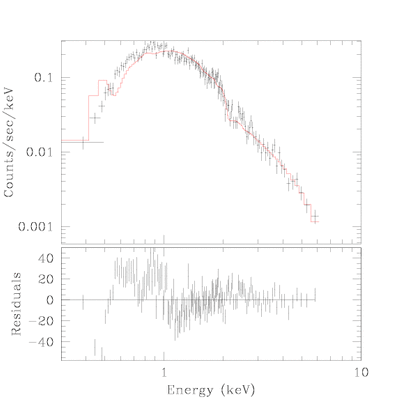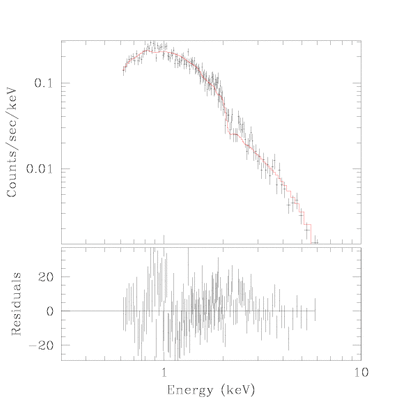True Color Image Gallery: Galactic SNR | Magellanic Cloud SNR

# Fit Detail for SNR J0526.0-6604

nH was dervide from central source.

## Central Object:

source=(xswabs * powlaw1d)
reduce chi^2 =
nh = 0.4528 10^22/cm^2
PARAMETERSDataSet 1: 329 data points -- 326 degrees of freedom.
• Statistic value = 384.565
• Probability [Q-value] = 0.014089
• Reduced statistic = 1.17965
--end--

## Point Source(2):

• spectum was re-fitted with ignoring energy below 0.6 keV.
• this nH value was used in estimating the flux value.
source=(xswabs * powlaw1d)
reduce chi^2 =
nh = 0.3391 10^22/cm^2
PARAMETERSDataSet 1: 317 data points -- 314 degrees of freedom.
• Statistic value = 174.925
• Probability [Q-value] = 1
• Reduced statistic = 0.557086
--end--

## Shell:

• spectrum from shell
• nH was fixed at the value derived above.
• two thermal plsma model.
source=(xswabs * (xsraymond + xsraymond))
reduce chi^2 =
nh = 0.3373 10^22/cm^2
PARAMETERSDataSet 4: 239 data points -- 235 degrees of freedom.
• Statistic value = 4711.76
• Probability [Q-value] = 0
• Reduced statistic = 20.05
--end--

## Total:

• spectrum from total
• nH and power-law component for point source was fixed at the derived value in the previous fit.
source=(xswabs * ((powlaw1d + xsraymond) + xsraymond))
reduce chi^2 =
nh = 0.3373 10^22/cm^2
PARAMETERSDataSet 3: 243 data points -- 239 degrees of freedom.
• Statistic value = 4725.87
• Probability [Q-value] = 0
• Reduced statistic = 19.7735
--end--

Back to SNR J0526.0-6604 IndexChandra Supernova Remnant CatalogMain Page | Galactic SNR | Magellanic Cloud SNRTrue Color Image Gallery: Galactic SNR | Magellanic Cloud SNR